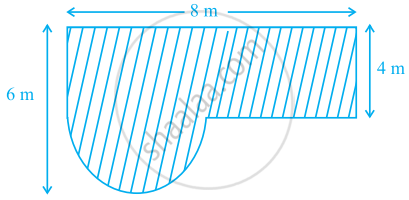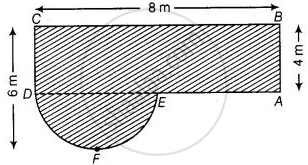# Find the area of the shaded field shown in figure. - Mathematics

Sum

Find the area of the shaded field shown in figure.#### Solution

In a figure, join EDFrom figure, radius of semi-circle DEF, r = 6 - 4 = 2 m

Now, area of rectangle ABCD = BC xx AB = 8 xx 4 = 32  cm^2

And area of semi-circle DFE = (pir^2)/2 = pi/2 (2)^2 = 2pi  m^2

∴ Area of shaded region = Area of rectangle ABCD + Area of semi-circle DFE

= (32 + 2pi)  m^2

Concept: Areas of Combinations of Plane Figures
Is there an error in this question or solution?

#### APPEARS IN

NCERT Mathematics Exemplar Class 10
Chapter 11 Area Related To Circles
Exercise 11.3 | Q 8 | Page 126
Share